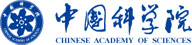Multimedia >>

## A 3 kHz High-Frequency Vibration Mirror Based on Resonant Mode

 Title: A 3 kHz High-Frequency Vibration Mirror Based on Resonant Mode Author(s): Han, WQ (Han, Weiqiang); Fan, ZJ (Fan, Zhenjie); Le, B (Le, Bai); Huang, T (Huang, Tao); Liu, B (Liu, Bo); Zhao, RJ (Zhao, Rujin); Gao, XD (Gao, Xiaodong) Source: ACTA MICROSCOPICA  Volume: 29  Issue: 4  Pages: 1866-1879  Published: 2020 Abstract: Infrared small/weak target detection systems detect small/weak target by subtracting the strong atmospheric background from the acquired image, in which a modulation process involving high-frequency vibration mirror (VM) is required. VM is also an indispensable core component in some laser scanning applications requiring extremely high accuracy. None of the existing VMs meet the requirements of large aperture and high frequency at the same time. This study deals with the development of a method to generate high-frequency vibration for large-aperture VMs, based on which a double torsion bar double moving magnet vibration mirror (DDVM) was designed. Two mathematical models based on DDVM, namely free vibration model and stimulated vibration model, are established, and then the conditions for achieving high frequency and large amplitude are obtained. Furthermore, a method for simulating and correcting the influence of the static magnetic attraction on the mirror surface shape is developed through a simulation of the designed DDVM based on the DDVM models, and a method for calculating the damping and driving force amplitude using the measured vibration bandwidth of DDVM structure is developed through dynamics simulation. Experimental results show that for a mirror with an optical aperture of Phi 40X56 mm, the optical scanning range reaches 0.584 degrees, and the vibration frequency reaches 3,033 Hz. The acceleration resulting from such vibration amplitude and frequency of the VM with the above aperture is more than 100 times the acceleration in rocket launch, which is difficult to achieve with other methods. The measured frequency is very close to the simulation result (3075 Hz) and the frequency calculated using the mathematical model (3098 Hz). The errors less than 2% prove the correctness of the DDVM mathematical model and the simulation analysis. From experimental data, the calculated bandwidth Delta f of DDVM is 6.1 Hz, the maximum gain coefficient Gn is 37694.7 rad.Hz2.N-1, and the damping coefficient D is 2.87X10-4 kg.m2.s-1. Thus, the accurate differential equation and the structure transfer function of DDVM are obtained. ISSN: 0798-4545 eISSN: 2224-8927Copyright © The Institute of Optics And Electronics, The chinese Academy of Sciences Address: Box 350, Shuangliu, Chengdu, Sichuan, China Email:dangban@ioe.ac.cn Post Code: 610 209 备案号：蜀ICP备05022581号